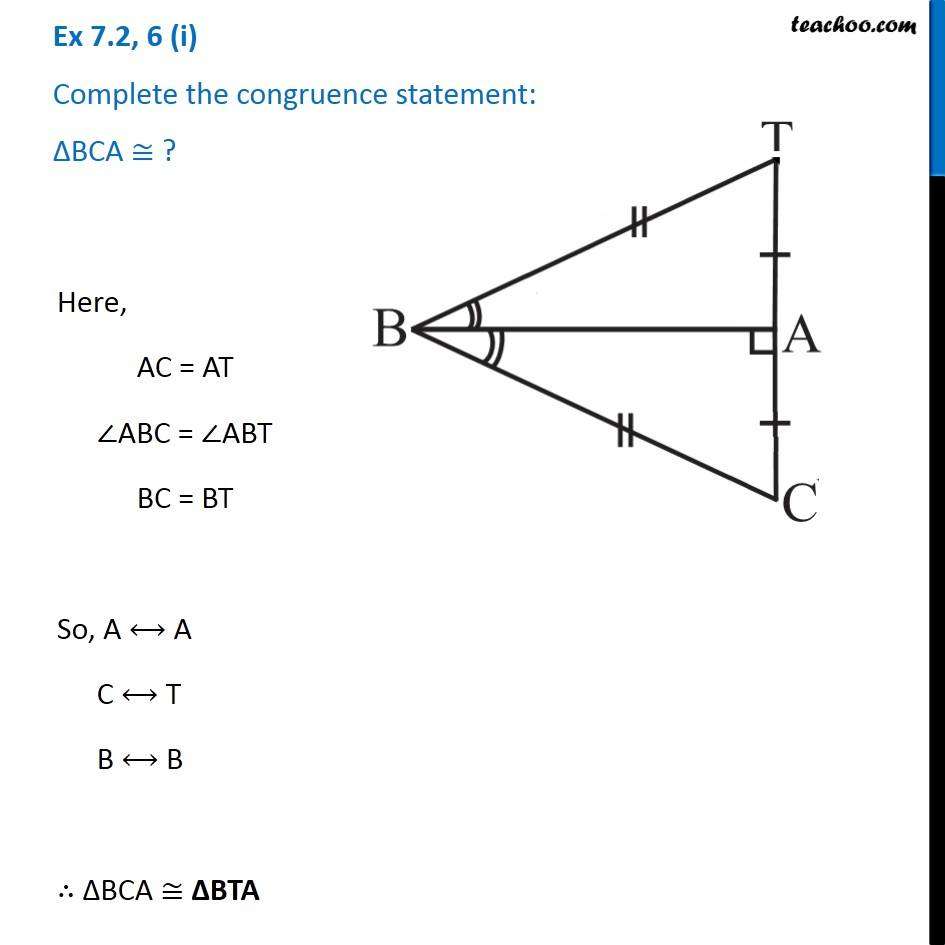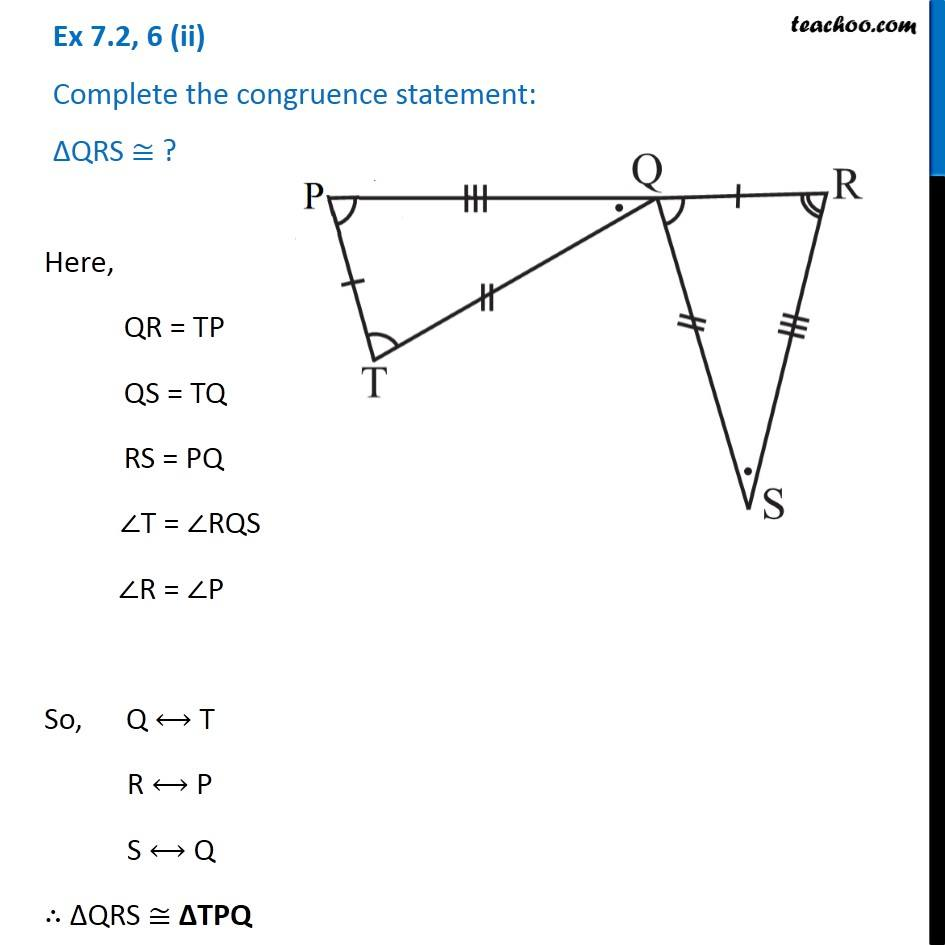Congruency - Different Criteria's

Congruence of Triangles
Serial order wiseLearn in your speed, with individual attention - Teachoo Maths 1-on-1 Class

### Transcript

Question 6 (i) Complete the congruence statement: ∆BCA ≅ ?Here, AC = AT ∠ABC = ∠ABT BC = BT So, A ⟷ A C ⟷ T B ⟷ B ∴ ∆BCA ≅ ∆BTA Question 6 (ii) Complete the congruence statement: ∆QRS ≅ ?Here, QR = TP QS = TQ RS = PQ ∠T = ∠RQS ∠R = ∠P So, Q ⟷ T R ⟷ P S ⟷ Q ∴ ∆QRS ≅ ∆TPQ